Name:    3rd Grade Basic Math Test 8

Multiple Choice
Identify the choice that best completes the statement or answers the question.

1.

Problem Solving – RIT 181 – 190

Find the missing numbers.
350, 355, 360, 365, ____, ____, ____
 a. 370, 380, 390 d. 370, 375, 380 b. 366, 367, 368 e. 364, 363, 362 c. 375, 385, 395

2.

It takes 50 beads to make a necklace. There are 10 beads in a package.
How many packages will you probably buy to make a necklace?
 a. one d. four b. two e. five c. three

3.

Tell how you would solve the problem. There are 8 cows in the field and 3 cows in the barn. How many more cows are in the field than in the barn?
 a. add c. multiply b. subtract d. divide

4.

Sam bought a baseball for \$6 and a mitt for \$14. How much did he
spend?
 a. \$21 d. \$8 b. \$18 e. \$20 c. \$10

5.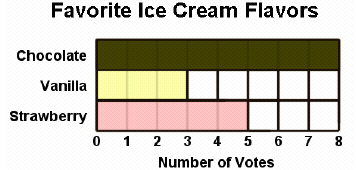How many more people chose chocolate than strawberry?
 a. 13 d. 3 b. 5 e. 2 c. 8

6.

17 - ___ = 9
 a. 8 d. 7 b. 6 e. 10 c. 9

7.

Paul and his father are flying to Mexico City. It costs \$495 for Paul’s father’s
ticket and \$328 for his own ticket. How much more does his father’s ticket cost?
 a. \$167 d. \$198 b. \$823 e. \$819 c. \$216

8.

The zoo has 54 parrots. The zookeeper wants to put 9 parrots in each cage.
How many cages will the zookeeper need?
 a. 6 d. 4 b. 9 e. 3 c. 7

9.

What information is needed to solve this problem?
Jill and her family are going to the circus. There are 2 adults and 3
children. They want to go on Saturday. Adult tickets cost \$4.25. How much will
it cost the family to get into the circus?
 a. The number of people in the family d. The cost of popcorn. b. How long they were at the circus. e. How far away they live from the circus. c. The cost of a child’s ticket.

10.

If you needed to give a friend directions to your home, which problem solving
strategy would be best?
 a. Draw a picture d. Work Backwards b. Guess and Check e. Find a Pattern c. Make a list

11.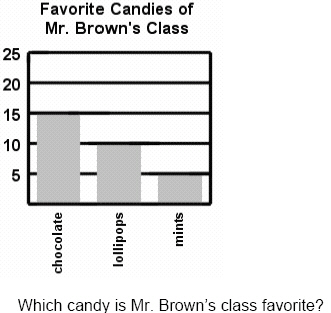a. No one likes mints. d. They like all candy. b. They like chocolate. e. Two children do not like candy. c. They like chocolate and lollipops.

12.

If Teresa has \$5.00 and buys a \$3.00 book, how much money will she have
left?
 a. \$8.00 d. \$5.00 b. \$1.00 e. \$3.00 c. \$2.00

13.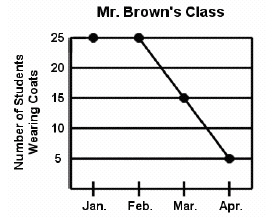During which 2 months did all of the students in Mr. Brown’s class wear a
coat?
 a. April was warmer d. January and February b. January and March e. The coldest months c. February and March

14.

Statistics/Probability and Graphing – Graphing – RIT 151 – 160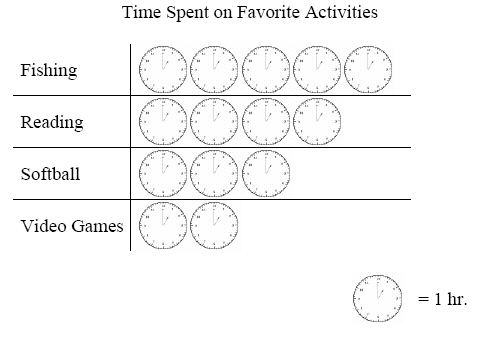How much more time is spent on fishing than reading?
 a. 1 d. 4 b. 2 e. 5 c. 3

15.

Statistics/Probability and Graphing – Graphing – RIT 161 – 170a. 6 d. 3 b. 5 e. 7 c. 4

16.

Statistics/Probability and Graphing – Probability and Prediction – RIT 161 -170

On which number is the spinner most likely to land?a. 1 d. 4 b. 2 e. 5 c. 3

17.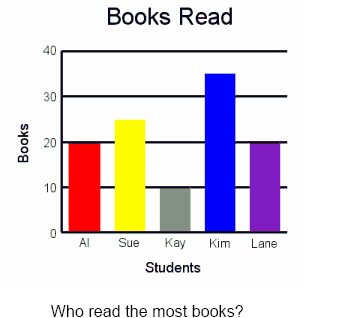a. Lane d. Kim b. Kay e. Al c. Sue

18.

Statistics/Probability and Graphing – Graphing – RIT 171 – 180a. 52 d. 25 b. 15 e. 5 c. 3

19.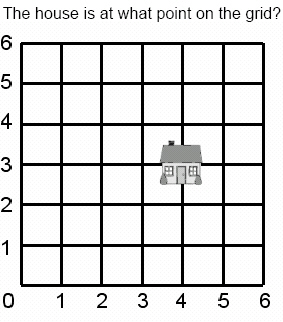a. (4,4) d. (3,2) b. (2,2) e. (3,4) c. (4,3)

20.a. Dog d. Horse b. Cat e. Fish c. Bird

21.

Statistics/Probability and Graphing – Probability and Prediction – RIT 171 –180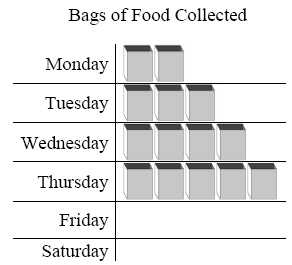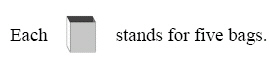How many bags do you think will be collected on Friday?
 a. 60 d. 30 b. 14 e. 25 c. 6

22.

Joan is making a quilt. She puts 4 stars in the first row, 5 stars in the second
row, and 4 stars in the third row.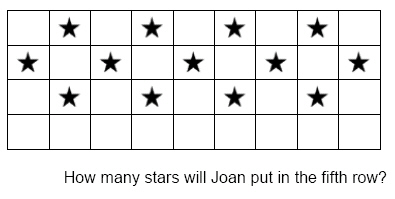a. 4 d. 3 b. 5 e. 13 c. 6

23.

Statistics/Probability and Graphing – Graphing – RIT 181 – 190

Mrs. Smith’s class did a survey to see which was the favorite color of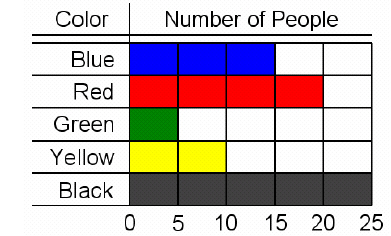Which color was the favorite?
 a. Red d. Blue b. Yellow e. Green c. Black

24.

Statistics/Probability and Graphing – Probability – RIT 181 – 190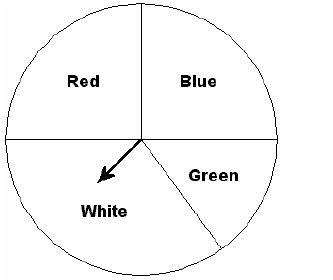What is the probability of the spinner stopping on yellow?
 a. possible d. impossible b. likely e. unlikely c. certain

25.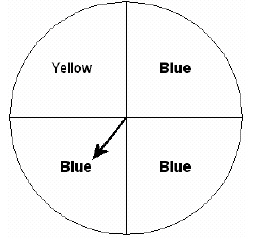What is the probability of the spinner stopping on blue?
 a. 1 to 3 d. 3 to 4 b. 5 to 2 e. 3 to 1 c. 1 to 2